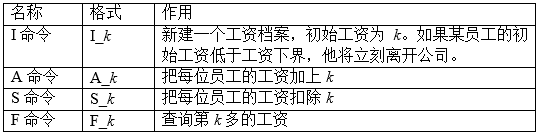# 刷题总结——郁闷的出纳员（bzoj1503）

## 题目：

NOI2004 DAY1 T1

### 题目描述

OIER 公司是一家大型专业化软件公司，有着数以万计的员工。作为一名出纳员，我的任务之一便是统计每位员工的工资。这本来是一份不错的工作，但是令人郁闷的是，我们的老板反复无常，经常调整员工的工资。如果他心情好，就可能把每位员工的工资加上一个相同的量。反之，如果心情不好，就可能把他们的工资扣除一个相同的量。我真不知道除了调工资他还做什么其它事情。

### 输入格式“下划线（ _ ）”表示一个空格，I 命令、A 命令、S 命令中的 k 是一个非负整数，F 命令中的 k 是一个正整数。

9 10
I 60
I 70
S 50
F 2
I 30
S 15
A 5
F 1
F 2

10
20
-1
2

### 备注

【数据范围】
I 命令的条数不超过 100000
A 命令和 S 命令的总条数不超过 100
F 命令的条数不超过 100000

【评分方法】

• 如果对于所有的 F 命令，你都输出了正确的答案，并且最后输出的离开公司的人数也是正确的，你将得到 10 分；
• 如果你只对所有的 F 命令输出了正确答案，得 6 分；
• 如果只有离开公司的人数是正确的，得 4 分；否则得 0 分。

# 仔细审题！！！！！

## 代码：

#include<iostream>
#include<cstdio>
#include<cstdlib>
#include<cmath>
#include<ctime>
#include<cctype>
#include<cstring>
#include<string>
#include<algorithm>
using namespace std;
const int N=3e5+5;
int val[N],father[N],cnt[N],son[N],size[N],root,tot;
int n,a,minn,totl=0,sum=0;
long long totcut=0;
char s;
inline int R()
{
char c;int f=0;
for(c=getchar();c<'0'||c>'9';c=getchar());
for(;c<='9'&&c>='0';c=getchar())
f=(f<<3)+(f<<1)+c-'0';
return f;
}
inline void clear(int now)
{
size[now]=son[now]=son[now]=val[now]=cnt[now]=father[now]=0;
}
inline void update(int now)
{
if(now)
{
size[now]=cnt[now];
if(son[now])  size[now]+=size[son[now]];
if(son[now])  size[now]+=size[son[now]];
}
}
inline int get(int a)
{
return son[father[a]]==a;
}
inline void rotate(int now)
{
int fa=father[now],ofa=father[fa],which=get(now);
son[fa][which]=son[now][which^1],father[son[fa][which]]=fa;
son[now][which^1]=fa,father[fa]=now,father[now]=ofa;
if(ofa)  son[ofa][son[ofa]==fa]=now;
update(fa),update(now);
}
inline void splay(int now)
{
while(father[now])
{
if(father[father[now]])  rotate(get(now)==get(father[now])?father[now]:now);
rotate(now);
}
root=now;
}
inline void insert(int x)
{
int now=root,last=0;
while(true)
{
if(!now)
{
now=++tot;size[now]=cnt[now]=1;father[now]=last;val[now]=x;
son[last][val[now]>val[last]]=now;update(last);splay(now);
break;
}
if(val[now]==x)
{
cnt[now]++;update(now);update(last);splay(now);
break;
}
last=now;now=son[now][x>val[now]];
}
}
inline int findx(int x)
{
int now=root;
while(true)
{
if(x<=size[son[now]])  now=son[now];
else
{
int temp=size[son[now]]+cnt[now];
if(x<=temp)  return val[now];
x-=temp;now=son[now];
}
}
}
inline int Delete()
{
int temp=size[son[root]]+cnt[root];int oldroot=root;
root=son[oldroot];father[root]=0;
clear(oldroot);
return temp;
}
int main()
{
//freopen("a.in","r",stdin);
n=R(),minn=R();
while(n--)
{
scanf("%s%d",s,&a);
if(s=='I')
{
if(a-sum>(minn-sum-1))
insert(a-sum),totl++;
}
if(s=='A')
sum+=a;
if(s=='S')
sum-=a;
if(s=='F')
{
if(totl<a)  cout<<"-1"<<endl;
else
{
int ans=findx(totl-a+1);
cout<<ans+sum<<endl;
}
}
int limit=minn-sum-1;insert(limit);
int temp=Delete();
totcut+=(long long)(temp-1);
totl=totl-temp+1;
}
cout<<totcut<<endl;
return 0;
}

posted @ 2017-09-07 22:06  AseanA  阅读(184)  评论(0编辑  收藏  举报